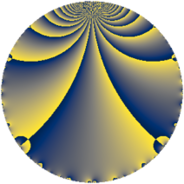# Properties

 Label 42.2.fLevel $42$ Weight $2$ Character orbit 42.f Rep. character $\chi_{42}(5,\cdot)$ Character field $\Q(\zeta_{6})$ Dimension $4$ Newform subspaces $1$ Sturm bound $16$ Trace bound $0$

# Learn more about

## Defining parameters

 Level: $$N$$ $$=$$ $$42 = 2 \cdot 3 \cdot 7$$ Weight: $$k$$ $$=$$ $$2$$ Character orbit: $$[\chi]$$ $$=$$ 42.f (of order $$6$$ and degree $$2$$) Character conductor: $$\operatorname{cond}(\chi)$$ $$=$$ $$21$$ Character field: $$\Q(\zeta_{6})$$ Newform subspaces: $$1$$ Sturm bound: $$16$$ Trace bound: $$0$$

## Dimensions

The following table gives the dimensions of various subspaces of $$M_{2}(42, [\chi])$$.

Total New Old
Modular forms 24 4 20
Cusp forms 8 4 4
Eisenstein series 16 0 16

## Trace form

 $$4q + 2q^{4} - 10q^{7} - 6q^{9} + O(q^{10})$$ $$4q + 2q^{4} - 10q^{7} - 6q^{9} - 6q^{10} + 12q^{15} - 2q^{16} + 12q^{19} + 12q^{22} + 6q^{24} + 4q^{25} - 2q^{28} - 6q^{31} - 18q^{33} - 12q^{36} + 4q^{37} - 6q^{40} - 6q^{42} - 32q^{43} - 12q^{46} + 22q^{49} + 12q^{51} + 12q^{52} + 18q^{54} + 6q^{58} + 6q^{60} + 24q^{63} - 4q^{64} - 4q^{67} + 18q^{70} + 24q^{73} - 24q^{78} + 2q^{79} - 18q^{81} - 24q^{82} - 24q^{85} - 18q^{87} + 6q^{88} - 12q^{91} - 24q^{94} + 6q^{96} + O(q^{100})$$

## Decomposition of $$S_{2}^{\mathrm{new}}(42, [\chi])$$ into newform subspaces

Label Dim. $$A$$ Field CM Traces $q$-expansion
$$a_2$$ $$a_3$$ $$a_5$$ $$a_7$$
42.2.f.a $$4$$ $$0.335$$ $$\Q(\zeta_{12})$$ None $$0$$ $$0$$ $$0$$ $$-10$$ $$q+\zeta_{12}q^{2}+(-\zeta_{12}-\zeta_{12}^{3})q^{3}+\zeta_{12}^{2}q^{4}+\cdots$$

## Decomposition of $$S_{2}^{\mathrm{old}}(42, [\chi])$$ into lower level spaces

$$S_{2}^{\mathrm{old}}(42, [\chi]) \cong$$ $$S_{2}^{\mathrm{new}}(21, [\chi])$$$$^{\oplus 2}$$